Skip to main content

### Control Systems in Python - Part 2 - Routh Hurwitz

In my last post Control Systems in Python Part 1, i described how to setup and use Python for doing some basic plotting of transfer functions. One of the biggest benefits of using sympy vs numeric packages like matlab/numpy/scipy is the fact that you can use symbolic variables. This post includes a function for computing the Routh Hurwitz table (Note: It does not work for row's of zeros).

Lets do my control systems design homework problem together :) (Warning: I have not verified if this answer is right so please correct me if it’s not!)

The Problem

The problem is DP 9.11 from Dorf & Bishop’s Modern Control Systems. ISBN 0136024580. Basically we have to design a controller to compensate for a system with a time delay.
The controller is: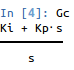And the system is: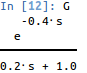First we approximate the exponential term with a 2nd order polynomial using pade(0.4,2) such that: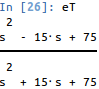Thus the approximated system is: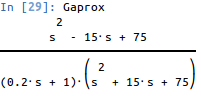Using frequency response methods, design the controller so that the overshoot of the system is P.O. <= 10%. From this we determine 0.59. When we plot the poles and zeros of the system we see that there are 3 poles in the LHP and two zeros in the RHP. The controller adds another pole at 0, so the zero of our controller should be somewhere to the left of the -5 pole on the real axis.
Thus Ki/Kp > 5. If Ki/Kp = 6. Using a Routh Hurwitz table we can see that 0<Kp<0.66 makes the system stable.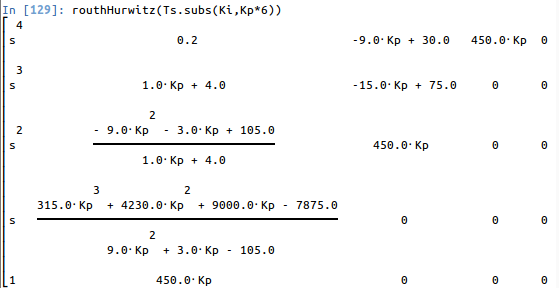The code for this function is:

def routhHurwitz(Ts,*args):
den = Poly(Ts.as_numer_denom(),s).all_coeffs()
n = len(den)-1
if n < 2:
return None
m = matrices.zeros(n+1)
# Insert the first two rows
c = [den[i] for i in range(0,n+1,2)]
for i in range(0,len(c)):
m[0,i] = c[i]
c = [den[i] for i in range(1,n+1,2)]
for i in range(0,len(c)):
m[1,i] = c[i]

# Compute the rest of the entries
for i in range(2,n+1):
for j in range(1,n):
if m[i-1,j] == 0:
m[i,j-1] = m[i-2,j]
elif m[i-1,0] !=0:
m[i,j-1] = ((m[i-1,0]*m[i-2,j]-m[i-2,0]*m[i-1,j])/m[i-1,0]).together().simplify()

m = m.col_insert(0, Matrix(([s**(n-i) for i in range(0,n+1)])))
return m

When we set Kp = 0.22 we get the controller: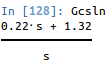giving the step response of: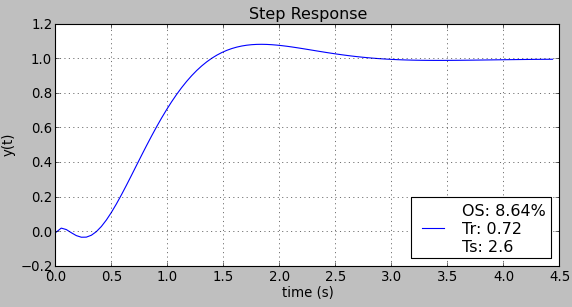if the gain is reduced the %OS reduces and Ts rises.

### A look at Chaquopy the Python SDK for Android

I recently heard about chaquopy - A "Python SDK for Android" and was really excited. There's already a demo app on the Play store for python 2 and python 3. As the author of enaml-native , I had to take a look to see how others are trying to tackle the python on android challenge.  So here's a short review and discussion of my initial thoughts on chaquopy. Demo I encourage you to try out the demo yourself to get a feel for it, but here's a short demo of what it does. Initial thoughts. It starts reasonably fast (about 3-4 seconds on my phone).  There's a noticeable delay when starting the python activity on my phone (much smoother on the emulator), but other than that the widgets look great and interaction is smooth! The apk is 11.54 MB and the app is only 18 MB installed (21MB if you include the cache), which is very good for bundling python! Also, the build process was a piece of cake!  I cloned the repo, opened in android studio, pressed play,

### Kivy vs React-Native for building cross platform mobile apps

I've built three apps now using Kivy and one with React-Native, just wanted to share my thoughts on both. Just a warning, I am strongly biased towards python and this is all based on opinion and experience and is thus worth what you pay for it. I don't claim to be an expert in either of these, just have worked with each for several months.  If something is incorrect I'd love to hear advice. Kivy Demo of one of the apps Pros: Nice to be able to run natively on the desktop WITHOUT a simulator Python is easy to work with Use (almost) any python library Very easy to create custom widgets Kivy properties and data binding just work. Way nicer than React's "state" / flux / redux whatever you want to call it (stupid?).  Native interfaces (pyjnius) and (pyobjc) Runs and feels pretty smooth Cons: Default widget toolkit looks like Android 4.4. Requiring you use your own widgets or a theming kit like KivyMD  if styling bothers you Creating dy

### Control Systems in Python - Part 1 - Bode and Step Response

I hate matlab with passion, yet sadly, nearly everyone uses it.  I'm a fan of Python and open source stuff so here's a simple article on how to do some common control systems stuff in Python. First we need to make sure the environment is setup. Install IPython (or you can use any other python shell, but a unicode supported shell is preferred) Install python-control (numpy, scipy) Install sympy These should do if your on Ubuntu/debian: sudo apt - get install python - sympy python-numpy python-scipy python-matplotlib ipython Then you need to install python control, see How to download and install python-control Intro to using Sympy Open ipython and run the following: import sympy from sympy import * sympy.init_printing() s = Symbol('s') Now we can do things like define transfer functions using the symbolic variable s. We can expand the bottom using the .simplify() method and we can do something more complex like... which is really nice because it